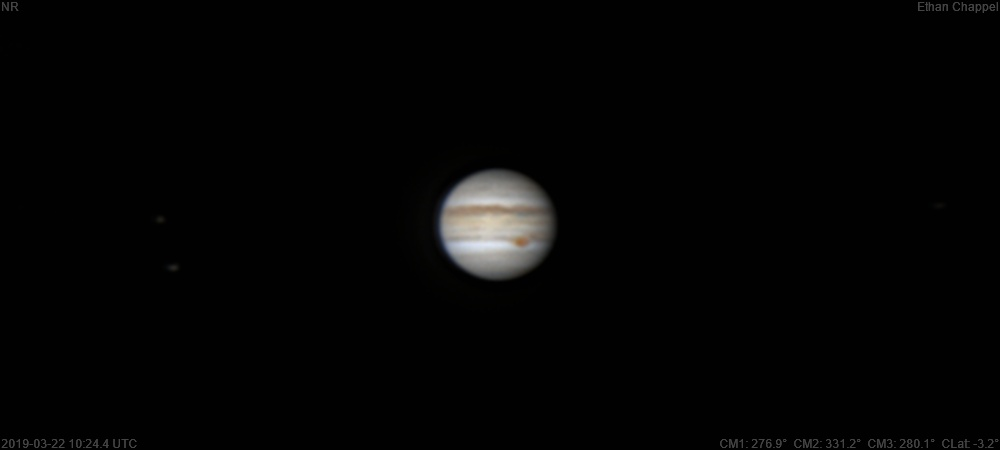Jupiter 2019-03-22 10:24 UTC

CM1: 276.90°

CM2: 331.20°

CM3: 280.10°

CLat: -3.20°

Description

I was getting anxious about having no access to a telescope five nights per week, so I decided to pack the 90mm Mak in the car and use it at my university. I attempted this in February, but my laptop powered down before I could capture anything because of the below-freezing temperature.

Notes:

• White Oval Z is just past the central meridian.
• The Equatorial Zone is still orange.
• The South Equatorial Belt to the west of the Great Red Spot is split by a white zone.

Equipment

ZWO ASI224MC

Orion Apex 90mm

Logs
```FireCapture v2.6  Settings
------------------------------------
Observer=Ethan Chappel
Camera=ZWO ASI224MC
Filter=RGB
Profile=Jupiter
Diameter=38.61"
Magnitude=-2.18
CMI=273.5° CMII=327.9° CMIII=276.8°  (during mid of capture)
FocalLength=1300mm (F/4)
Resolution=0.60"
Filename=2019-03-22-1019_9-EC-RGB-Jup.ser
Date=2019_03_22
Start=10_18_40.915
Mid=10_19_55.938
End=10_21_10.961
Start(UT)=10_18_40.915
Mid(UT)=10_19_55.938
End(UT)=10_21_10.961
Duration=150.046s
Date_format=yyyy_MM_dd
Time_format=HH_mm_ss
LT=UT -6h
Frames captured=3751
File type=SER
Binning=no
Bit depth=8bit
Debayer=yes
DebayerAlgorithm=NearestNeighbor
BayerPattern=RG
ROI=800x800
ROI(Offset)=8x8
FPS (avg.)=24
Shutter=40.00ms
Gain=220 (36%)
Gamma=50 (off)
FPS=100 (off)
HighSpeed=off
AutoHisto=75 (off)
HardwareBin=off
Brightness=1 (off)
WRed=55 (off)
SoftwareGain=10 (off)
AutoGain=off
WBlue=95 (off)
USBTraffic=100 (off)
AutoExposure=off
Histogramm(min)=0
Histogramm(max)=75
Histogramm=29%
Noise(avg.deviation)=0.06
AutoAlign=false
PreFilter=none
Limit=150 Seconds
Sensor temperature=23.2°C
FireCapture v2.6  Settings
------------------------------------
Observer=Ethan Chappel
Camera=ZWO ASI224MC
Filter=RGB
Profile=Jupiter
Diameter=38.61"
Magnitude=-2.18
CMI=279.0° CMII=333.3° CMIII=282.3°  (during mid of capture)
FocalLength=1300mm (F/4)
Resolution=0.59"
Filename=2019-03-22-1028_9-EC-RGB-Jup.ser
Date=2019_03_22
Start=10_27_40.331
Mid=10_28_55.341
End=10_30_10.351
Start(UT)=10_27_40.331
Mid(UT)=10_28_55.341
End(UT)=10_30_10.351
Duration=150.020s
Date_format=yyyy_MM_dd
Time_format=HH_mm_ss
LT=UT -6h
Frames captured=3641
File type=SER
Binning=no
Bit depth=8bit
Debayer=yes
DebayerAlgorithm=NearestNeighbor
BayerPattern=RG
ROI=800x800
ROI(Offset)=8x8
FPS (avg.)=24
Shutter=40.00ms
Gain=220 (36%)
Gamma=50 (off)
FPS=100 (off)
HighSpeed=off
AutoHisto=75 (off)
HardwareBin=off
Brightness=1 (off)
WRed=55 (off)
SoftwareGain=10 (off)
AutoGain=off
WBlue=95 (off)
USBTraffic=100 (off)
AutoExposure=off
Histogramm(min)=0
Histogramm(max)=97
Histogramm=38%
Noise(avg.deviation)=0.06
AutoAlign=false
PreFilter=none
Limit=150 Seconds
Sensor temperature=21.2°C
```